## RD Sharma Class 9 Solutions Chapter 3 Rationalisation Ex 3.2

These Solutions are part of RD Sharma Class 9 Solutions. Here we have given RD Sharma Class 9 Solutions Chapter 3 Rationalisation Ex 3.2

Question 1.
Rationalise the denominators of each of the following(i – vii):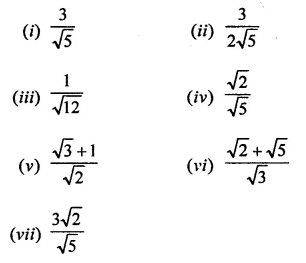>
Solution: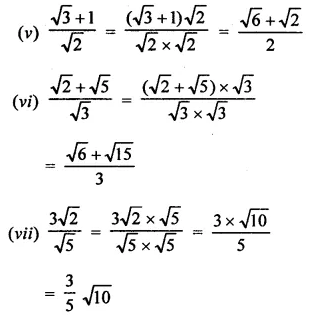Question 2.
Find the value to three places of decimals of each of the following. It is given that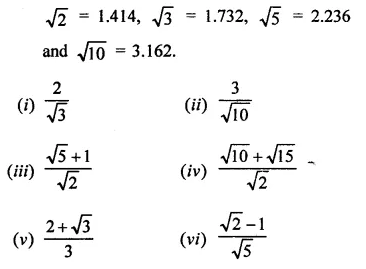Solution: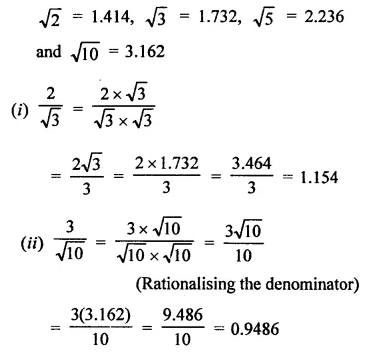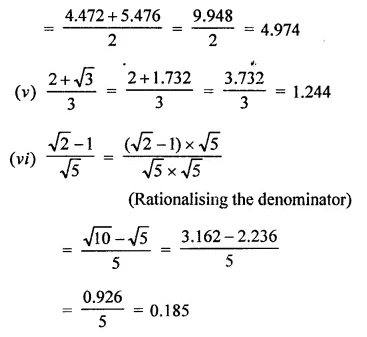Question 3.
Express each one of the following with rational denominator:Solution: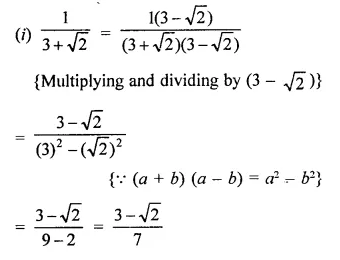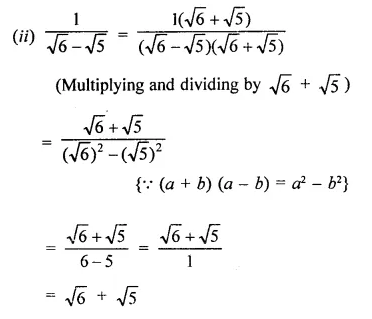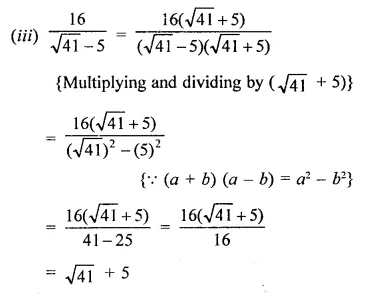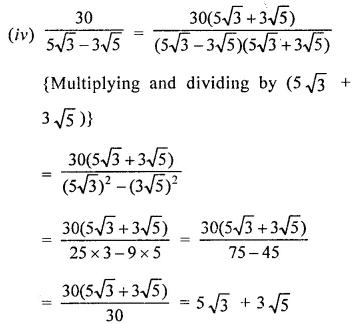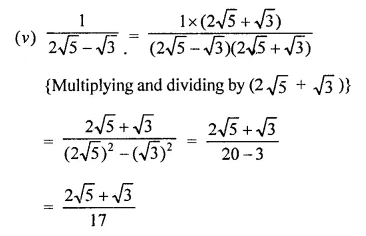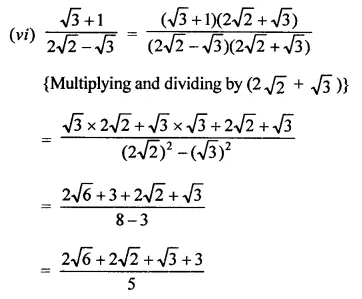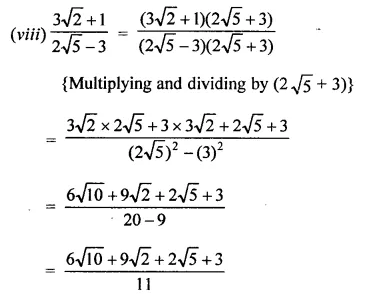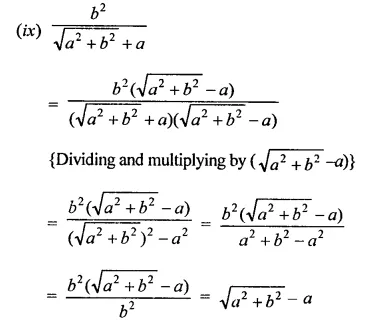Question 4.
Rationales the denominator and simplify:Solution: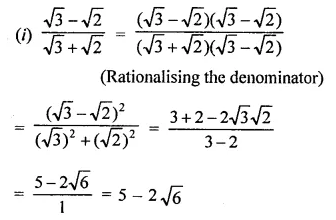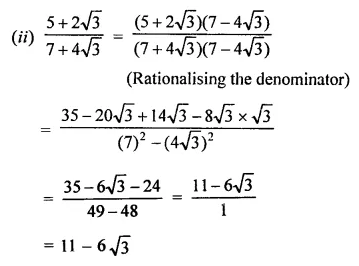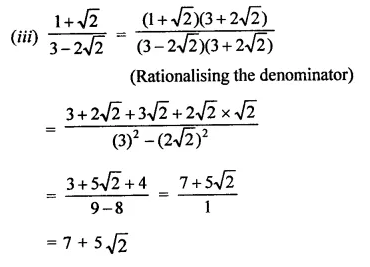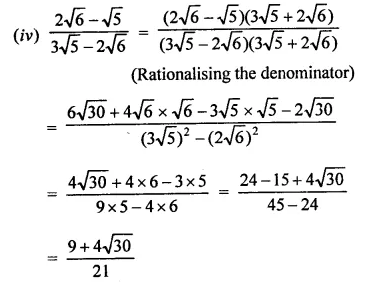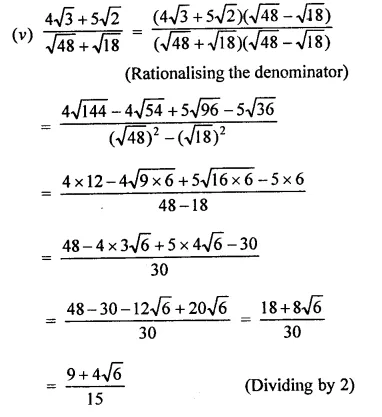Question 5.
Simplify:Solution: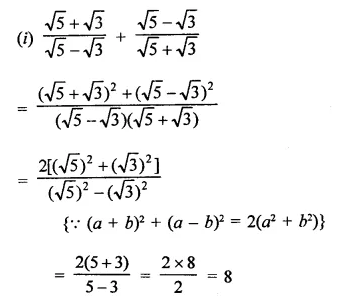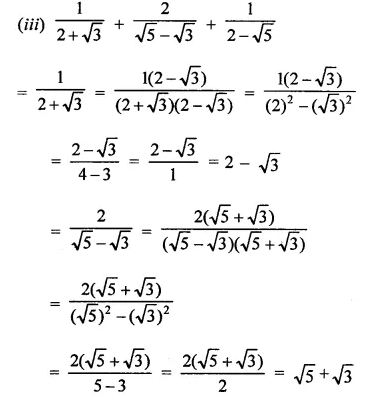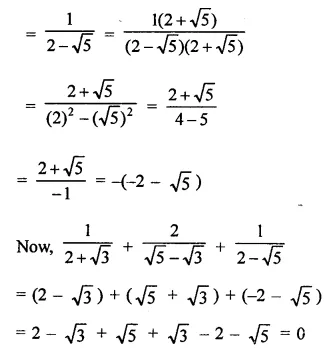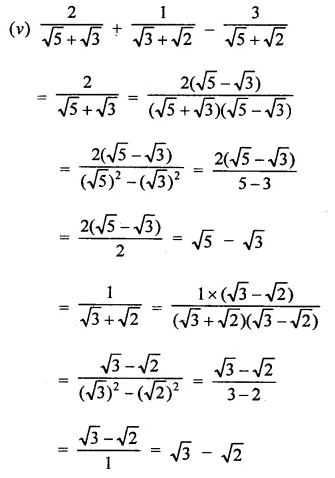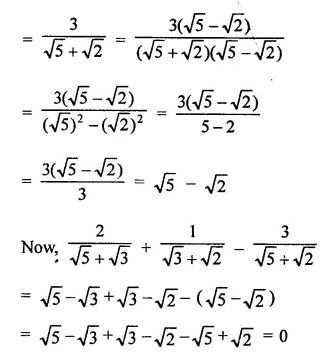Question 6.
In each of the following determine rational numbers a and b:Solution: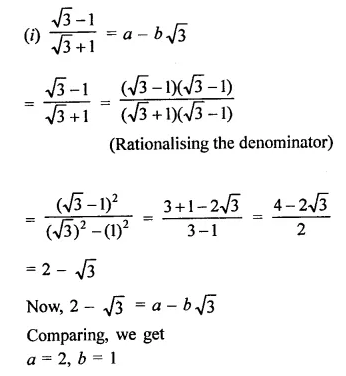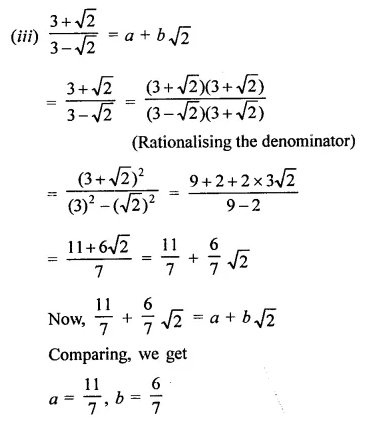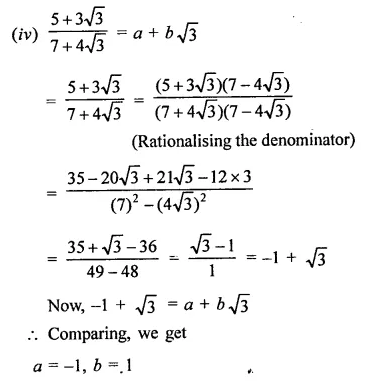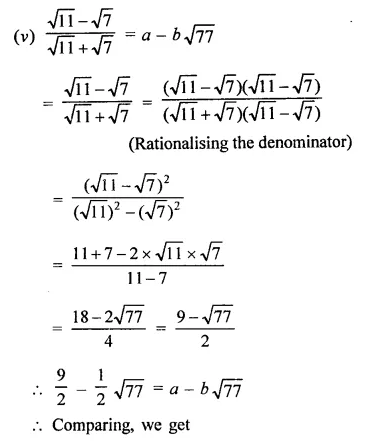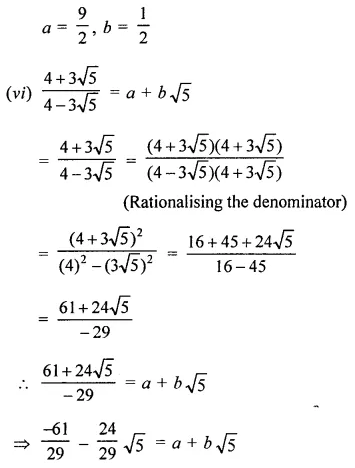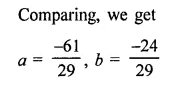Question 7.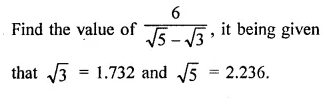Solution: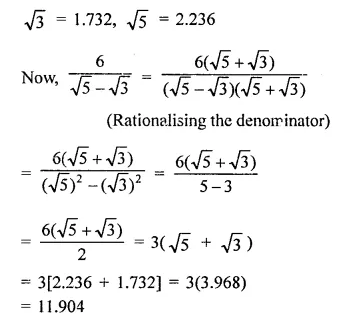Question 8.
Find the values of each of the following correct to three places of decimals, it being given that $$\sqrt { 2 }$$  = 1.4142, $$\sqrt { 3 }$$ = 1-732, $$\sqrt { 5 }$$  = 2.2360, $$\sqrt { 6 }$$ =  2.4495 and $$\sqrt { 10 }$$  = 3.162.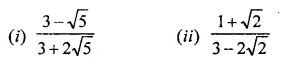Solution: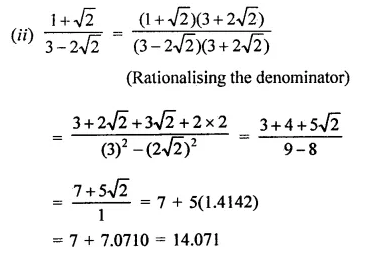Question 9.
Simplify:Solution: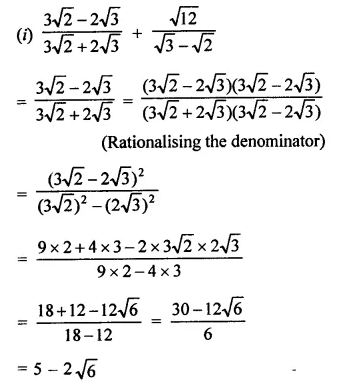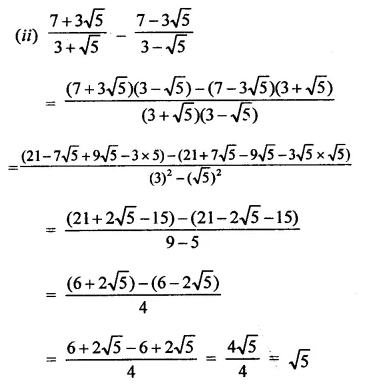Question 10.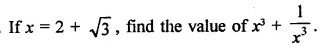Solution: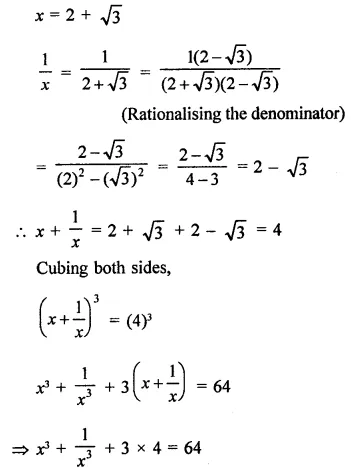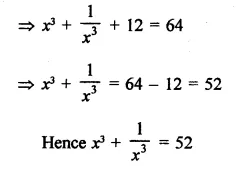Question 11.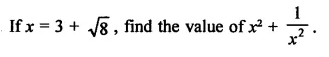Solution: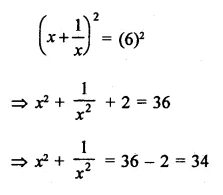Question 12.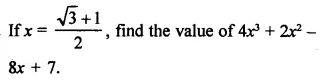Solution: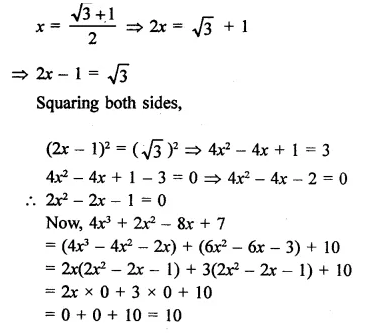Hope given RD Sharma Class 9 Solutions Chapter 3 Rationalisation Ex 3.2 are helpful to complete your math homework.

If you have any doubts, please comment below. Learn Insta try to provide online math tutoring for you.